To Top

ctr模型汇总

## FM

$\phi(x) = w_0+\sum _{i}w_ix_i+\sum_{i}\sum_{j<i}w_{ij}x_ix_j$

FM模型：

$\hat{y} = w_0+\sum _{i=1}^nw_ix_i+\sum_{i=1}^{n-1}\sum_{j=i+1}^{n}\left \langle \mathbf{v}_i,\mathbf{v}_j \right \rangle x_ix_j$

• $$w_0\in \mathbb{R}$$
• $$\mathbf{w}\in \mathbb{R}^n$$
• $$\mathbf{V}\in \mathbb{R}^{n\times k}$$
• $$\hat{w_{ij}}=\mathbf{v}_i\mathbf{v}_j^T=\sum _{l=1}^kv_{il}v_{jl}$$ 所以上式中的$$\mathbf{v}_i$$就表示$$\mathbf{V}$$这个矩阵的第i行（有k列），而$$\left \langle \mathbf{v}_i,\mathbf{v}_j \right \rangle$$就表示第i行和和j行这两个向量的内积（得到一个数），而得到的正好就是权重矩阵的第i行第j列的元素$$\hat{w}_{ij}$$，而$$\hat{w}$$这个矩阵是$$(n-1)\times(n-1)$$维的矩阵，刻画的是相邻两个x【$$x_i$$$$x_{i+1}$$】之间的系数。因此，可以理解为，将这个$$(n-1)\times(n-1)$$维的矩阵用一个$$n\times k$$维的低秩矩阵来表示。

$\sum ^n_{i=1}\sum ^n_{j=i+1}x_ix_j=\frac{1}{2}[(\sum ^n_{i=1}x_i)^2-\sum^n_{i=1}x_i^2]$

$\sum xxv=\frac{1}{2}[\sum (xv)^2-\sum x^2v^2]$

tf实现：

class FM(Model):
def __init__(self, input_dim=None, output_dim=1, factor_order=10, init_path=None, opt_algo='gd', learning_rate=1e-2,
l2_w=0, l2_v=0, random_seed=None):
Model.__init__(self)
# 一次、二次交叉、偏置项
init_vars = [('w', [input_dim, output_dim], 'xavier', dtype),
('v', [input_dim, factor_order], 'xavier', dtype),
('b', [output_dim], 'zero', dtype)]
self.graph = tf.Graph()
with self.graph.as_default():
if random_seed is not None:
tf.set_random_seed(random_seed)
self.X = tf.sparse_placeholder(dtype)
self.y = tf.placeholder(dtype)
self.vars = init_var_map(init_vars, init_path)

w = self.vars['w']
v = self.vars['v']
b = self.vars['b']

# [(x1+x2+x3)^2 - (x1^2+x2^2+x3^2)]/2
# 先计算所有的交叉项，再减去平方项(自己和自己相乘)
X_square = tf.SparseTensor(self.X.indices, tf.square(self.X.values), tf.to_int64(tf.shape(self.X)))
xv = tf.square(tf.sparse_tensor_dense_matmul(self.X, v))
p = 0.5 * tf.reshape(
tf.reduce_sum(xv - tf.sparse_tensor_dense_matmul(X_square, tf.square(v)), 1),
[-1, output_dim])
xw = tf.sparse_tensor_dense_matmul(self.X, w)
logits = tf.reshape(xw + b + p, [-1])
self.y_prob = tf.sigmoid(logits)

self.loss = tf.reduce_mean(
tf.nn.sigmoid_cross_entropy_with_logits(logits=logits, labels=self.y)) + \
l2_w * tf.nn.l2_loss(xw) + \
l2_v * tf.nn.l2_loss(xv)
self.optimizer = get_optimizer(opt_algo, learning_rate, self.loss)

#GPU设定
config = tf.ConfigProto()
config.gpu_options.allow_growth = True
self.sess = tf.Session(config=config)
# 图中所有variable初始化
tf.global_variables_initializer().run(session=self.sess)


$\hat{y} = w_0+\left \langle w,x \right \rangle + \left \langle xV,xV \right \rangle$

• 先对离散特征进行embedding
• 然后对embedding后的向量进行内积来做二阶特征组合

## FFM

Field-aware Factorization Machines for CTR Prediction

FFM把相同性质的特征归于同一个field。每一维特征$$x_i$$，针对其他特征的每一种field $$f_j$$，都会学习一个隐向量$$V_{i,f_j}$$。所以隐向量不仅与特征有关，也与field相关。假设有$$n$$个特征，$$f$$个field，那么FFM的二次项总共有$$nf$$个隐向量，而FM中，每一维特征的隐向量只有一个。公式如下：

$y=w_0+\sum^n_{i=1}w_ix_i+\sum^n_{i=1}\sum ^n_{j=i+1} \left \langle V_{i,f_j}, V_{j,f_i} \right \rangle x_i x_j$

FFM做召回可以参考：推荐系统召回四模型之二：沉重的FFM模型

“微博在业务中的实践表明，如果采取FM召回模型，对于有些应用来说，一阶项对于最终效果有明显影响，所以在用FM/FFM做召回的时候，是需要将一阶项考虑进去的，这可能是个别一阶特征比较重要导致的。我们在Criteo数据集合的实验结果也证明：如果是FM模型，一阶项是有用的，去掉一阶项，只保留二阶项，AUC大约会掉1个绝对百分点，对于CTR来说，这个差距还是很明显的；而如果是采用DeepFM模型，则FM部分是否保留一阶项对最终结果没有什么影响，这说明DNN的隐层有效地将一阶项的作用吸收掉了。”

## FNN, SNN

Deep Learning over Multi-field Categorical Data - A Case Study on User Response Prediction in Display Ads

### FNN

FM与MLP进行了结合。它有着十分显著的特点：

• 采用FM预训练得到的隐含层及其权重作为神经网络的第一层的初始值，之后再不断堆叠全连接层，最终输出预测的点击率。
• 可以将FNN理解成一种特殊的embedding+MLP，其要求第一层嵌入后的各领域特征维度一致，并且emb权重的初始化是FM预训练好的。
• 不是一个端到端的训练过程，有贪心预训练的思路。而且如果不考虑预训练过程，模型网络结构也没有考虑低阶特征组合。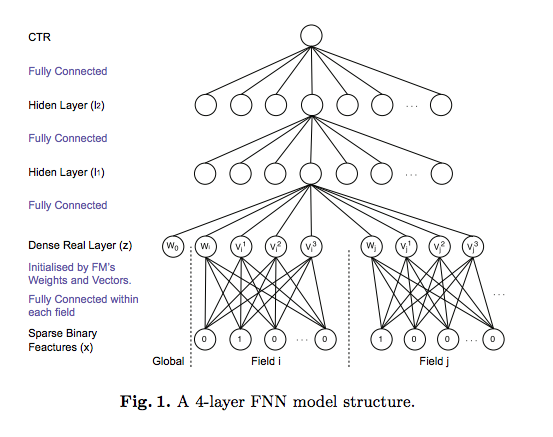tf代码：

class FNN(Model):
def __init__(self, field_sizes=None, embed_size=10, layer_sizes=None, layer_acts=None, drop_out=None,
embed_l2=None, layer_l2=None, init_path=None, opt_algo='gd', learning_rate=1e-2, random_seed=None):
Model.__init__(self)
init_vars = []
num_inputs = len(field_sizes)
for i in range(num_inputs):
init_vars.append(('embed_%d' % i, [field_sizes[i], embed_size], 'xavier', dtype))
node_in = num_inputs * embed_size
for i in range(len(layer_sizes)):
init_vars.append(('w%d' % i, [node_in, layer_sizes[i]], 'xavier', dtype))
init_vars.append(('b%d' % i, [layer_sizes[i]], 'zero', dtype))
node_in = layer_sizes[i]
self.graph = tf.Graph()
with self.graph.as_default():
if random_seed is not None:
tf.set_random_seed(random_seed)
self.X = [tf.sparse_placeholder(dtype) for i in range(num_inputs)]
self.y = tf.placeholder(dtype)
self.keep_prob_train = 1 - np.array(drop_out)
self.keep_prob_test = np.ones_like(drop_out)
self.layer_keeps = tf.placeholder(dtype)
self.vars = init_var_map(init_vars, init_path)
w0 = [self.vars['embed_%d' % i] for i in range(num_inputs)]
xw = tf.concat([tf.sparse_tensor_dense_matmul(self.X[i], w0[i]) for i in range(num_inputs)], 1)
l = xw

#全连接部分
for i in range(len(layer_sizes)):
wi = self.vars['w%d' % i]
bi = self.vars['b%d' % i]
print(l.shape, wi.shape, bi.shape)
l = tf.nn.dropout(
activate(
tf.matmul(l, wi) + bi,
layer_acts[i]),
self.layer_keeps[i])

l = tf.squeeze(l)
self.y_prob = tf.sigmoid(l)

self.loss = tf.reduce_mean(
tf.nn.sigmoid_cross_entropy_with_logits(logits=l, labels=self.y))
if layer_l2 is not None:
self.loss += embed_l2 * tf.nn.l2_loss(xw)
for i in range(len(layer_sizes)):
wi = self.vars['w%d' % i]
self.loss += layer_l2[i] * tf.nn.l2_loss(wi)
self.optimizer = get_optimizer(opt_algo, learning_rate, self.loss)

config = tf.ConfigProto()
config.gpu_options.allow_growth = True
self.sess = tf.Session(config=config)
tf.global_variables_initializer().run(session=self.sess)


### SNN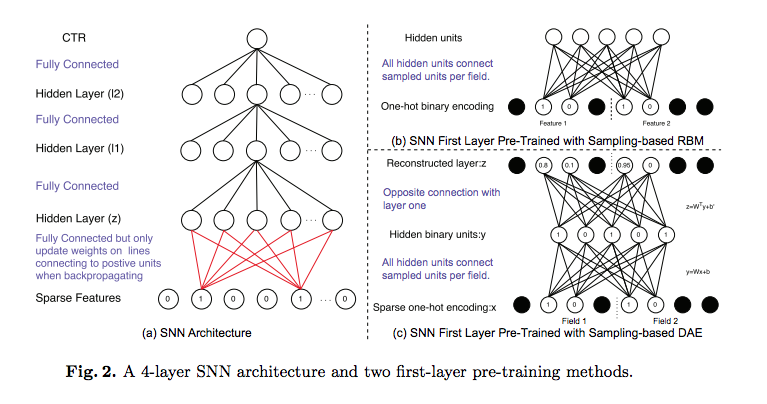FNN比SNN-DAE和SNN-RBM好，两种SNN结果总是差不多，但都比LR和FM好。

## NFM

Neural Factorization Machines for Sparse Predictive Analytics

NFM的基本特点：

• 利用二阶交互池化层（Bi-Interaction Pooling）对FM嵌入后的向量两两进行元素级别的乘法，形成同维度的向量求和后作为前馈神经网络的输入。
• NFM与DeepFM的区别是没有单独的FM的浅层网络进行联合训练，而是将其整合后直接输出给前馈神经网络
• 当MLP的全连接层都是恒等变换且最后一层参数全为1时，NFM就退化成了FM。可见，NFM是FM的推广，它推迟了FM的实现过程，并在其中加入了更多非线性运算。
• NFM与FNN非常相似。它们的主要区别是NFM在embedding之后对特征进行了两两逐元素乘法。因为逐元素相乘的向量维数不变，之后对这些向量求和的维数仍然与embedding的维数一致。因此输入到MLP的参数比起直接concatenate的FNN更少。

tf实现：

def model_fn(features, labels, mode, params):
"""Bulid Model function f(x) for Estimator."""
#------hyperparameters----
field_size = params["field_size"]
feature_size = params["feature_size"]
embedding_size = params["embedding_size"]
l2_reg = params["l2_reg"]
learning_rate = params["learning_rate"]
#optimizer = params["optimizer"]
layers = map(int, params["deep_layers"].split(','))
dropout = map(float, params["dropout"].split(','))

#------bulid weights------
Global_Bias = tf.get_variable(name='bias', shape=, initializer=tf.constant_initializer(0.0))
Feat_Bias = tf.get_variable(name='linear', shape=[feature_size], initializer=tf.glorot_normal_initializer())
Feat_Emb = tf.get_variable(name='emb', shape=[feature_size,embedding_size], initializer=tf.glorot_normal_initializer())

#------build feaure-------
feat_ids  = features['feat_ids']
feat_ids = tf.reshape(feat_ids,shape=[-1,field_size])
feat_vals = features['feat_vals']
feat_vals = tf.reshape(feat_vals,shape=[-1,field_size])

#------build f(x)------
with tf.variable_scope("Linear-part"):
feat_wgts = tf.nn.embedding_lookup(Feat_Bias, feat_ids)         # None * F * 1
y_linear = tf.reduce_sum(tf.multiply(feat_wgts, feat_vals),1)

with tf.variable_scope("BiInter-part"):
embeddings = tf.nn.embedding_lookup(Feat_Emb, feat_ids)         # None * F * K
feat_vals = tf.reshape(feat_vals, shape=[-1, field_size, 1])
embeddings = tf.multiply(embeddings, feat_vals)                 # vij * xi
sum_square_emb = tf.square(tf.reduce_sum(embeddings,1))
square_sum_emb = tf.reduce_sum(tf.square(embeddings),1)
deep_inputs = 0.5*tf.subtract(sum_square_emb, square_sum_emb)   # None * K

with tf.variable_scope("Deep-part"):
if mode == tf.estimator.ModeKeys.TRAIN:
train_phase = True
else:
train_phase = False

if mode == tf.estimator.ModeKeys.TRAIN:
deep_inputs = tf.nn.dropout(deep_inputs, keep_prob=dropout)                      # None * K
for i in range(len(layers)):
deep_inputs = tf.contrib.layers.fully_connected(inputs=deep_inputs, num_outputs=layers[i], \
weights_regularizer=tf.contrib.layers.l2_regularizer(l2_reg), scope='mlp%d' % i)

if FLAGS.batch_norm:
deep_inputs = batch_norm_layer(deep_inputs, train_phase=train_phase, scope_bn='bn_%d' %i)   #放在RELU之后 https://github.com/ducha-aiki/caffenet-benchmark/blob/master/batchnorm.md#bn----before-or-after-relu
if mode == tf.estimator.ModeKeys.TRAIN:
deep_inputs = tf.nn.dropout(deep_inputs, keep_prob=dropout[i])                              #Apply Dropout after all BN layers and set dropout=0.8(drop_ratio=0.2)
#deep_inputs = tf.layers.dropout(inputs=deep_inputs, rate=dropout[i], training=mode == tf.estimator.ModeKeys.TRAIN)

y_deep = tf.contrib.layers.fully_connected(inputs=deep_inputs, num_outputs=1, activation_fn=tf.identity, \
weights_regularizer=tf.contrib.layers.l2_regularizer(l2_reg), scope='deep_out')
y_d = tf.reshape(y_deep,shape=[-1])

with tf.variable_scope("NFM-out"):
#y_bias = Global_Bias * tf.ones_like(labels, dtype=tf.float32)  # None * 1  warning;这里不能用label，否则调用predict/export函数会出错，train/evaluate正常；初步判断estimator做了优化，用不到label时不传
y_bias = Global_Bias * tf.ones_like(y_d, dtype=tf.float32)      # None * 1
y = y_bias + y_linear + y_d
pred = tf.sigmoid(y)

predictions={"prob": pred}
export_outputs = {tf.saved_model.signature_constants.DEFAULT_SERVING_SIGNATURE_DEF_KEY: tf.estimator.export.PredictOutput(predictions)}
# Provide an estimator spec for ModeKeys.PREDICT
if mode == tf.estimator.ModeKeys.PREDICT:
return tf.estimator.EstimatorSpec(
mode=mode,
predictions=predictions,
export_outputs=export_outputs)

#------bulid loss------
loss = tf.reduce_mean(tf.nn.sigmoid_cross_entropy_with_logits(logits=y, labels=labels)) + \
l2_reg * tf.nn.l2_loss(Feat_Bias) + l2_reg * tf.nn.l2_loss(Feat_Emb)

# Provide an estimator spec for ModeKeys.EVAL
eval_metric_ops = {
"auc": tf.metrics.auc(labels, pred)
}
if mode == tf.estimator.ModeKeys.EVAL:
return tf.estimator.EstimatorSpec(
mode=mode,
predictions=predictions,
loss=loss,
eval_metric_ops=eval_metric_ops)

#------bulid optimizer------
optimizer = tf.train.AdamOptimizer(learning_rate=learning_rate, beta1=0.9, beta2=0.999, epsilon=1e-8)
elif FLAGS.optimizer == 'Momentum':
optimizer = tf.train.MomentumOptimizer(learning_rate=learning_rate, momentum=0.95)
elif FLAGS.optimizer == 'ftrl':
optimizer = tf.train.FtrlOptimizer(learning_rate)

train_op = optimizer.minimize(loss, global_step=tf.train.get_global_step())

# Provide an estimator spec for ModeKeys.TRAIN modes
if mode == tf.estimator.ModeKeys.TRAIN:
return tf.estimator.EstimatorSpec(
mode=mode,
predictions=predictions,
loss=loss,
train_op=train_op)


## AFM

Attentional Factorization Machines:Learning theWeight of Feature Interactions via Attention Networks

NFM的主要创新点是在FM过程中添加了逐元素相乘的运算来增加模型的复杂度。但没有在此基础上添加更复杂的运算过程，比如对加权求和。

AFM的特点是：

• AFM与NFM都是致力于充分利用二阶特征组合的信息，对嵌入后的向量两两进行逐元素乘法，形成同维度的向量。而且AFM没有MLP部分
• AFM通过在逐元素乘法之后形成的向量进行加权求和，而且权重是基于网络自身来产生的。其方法是引入一个注意力子网络（Attention Net）。
• 权重都相等时，AFM退化成无全连接层的NFM
• “注意力子网络”的主要操作是进行矩阵乘法，其最终输出结果为softmax，以保证各分量的权重本身是一个概率分布。

## PNN

Product-based Neural Networks for User Response Prediction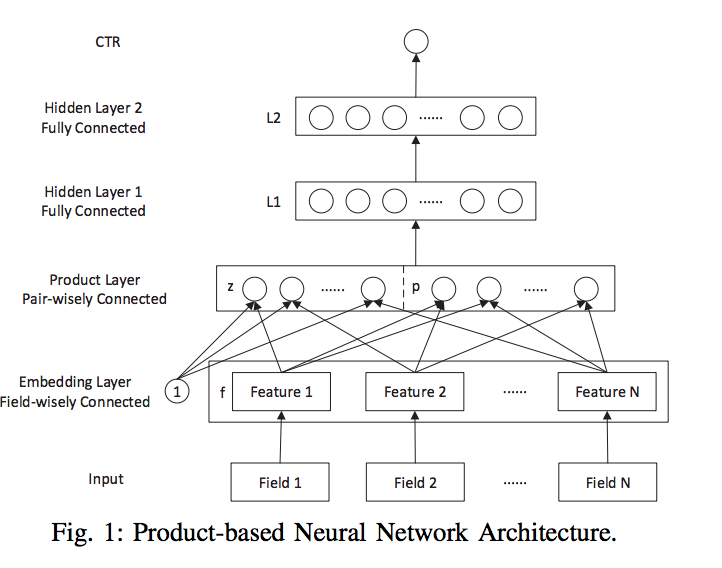• 首先对输入数据进行embedding处理，得到一个low-dimensional vector层
• 对该层的任意两个feature进行内积或是外积处理就得到上图的蓝色节点，
• 是把这些Feature直接和1相乘复制到上一层的Z中，
• 然后把Z和P接在一起就可以作为神经网络的输入层，
• 在此基础上我们就可以应用神经网络去模型了。

### IPNN

field之间使用内积。左边的z就是一个NxM维的，而对于p来讲，p是NxN的。所以对p来讲，使用矩阵分解来简化问题：

$W^n_p\odot p=\sum ^N_{i=1}\sum ^N_{j=1}\left \langle \theta ^i_n,\theta ^j_n \right \rangle \left \langle f_i,f_j \right \rangle$

### PNN小结

• 利用二阶向量积层（Pair-wisely Connected Product Layer）对FM嵌入后的向量两两进行向量积，形成的结果作为之后MLP的输入。PNN采用的向量积有内积与外积两种形式。
• PNN中向量与常数1进行的乘法运算其实与FNN类似，不是PNN的主要创新点。
• 对于内积形式的PNN，因为两个向量相乘的结果为标量，可以直接把各个标量“拼接”成一个大向量，就可以作为MLP的输入了。
• 当MLP的全连接层都是恒等变换且最后一层参数全为1时，内积形式的PNN就退化成了FM。
• 对于外积形式的PNN，因为两个向量相乘相当于列向量与行向量进行矩阵相乘，得到的结果为一个矩阵。各个矩阵向之前内积形式的操作一样直接拼接起来维数太多，论文的简化方案是直接对各个矩阵进行求和，得到的新矩阵（可以理解成之后对其拉长成向量）就直接作为MLP的输入。
• 观察计算图发现外积形式的PNN与NFM很像，其实就是PNN把NFM的逐元素乘法换成了外积。

## CCPM

CIKM2015的文章A Convolutional Click Prediction Model

• 在单条广告展示中（single ad impression），包括许多元素：element = (user, query, ad, impression time, site category, device type, etc)。用户是否点击一个广告与用户的历史ad impression有关。

ccpm包括convolutional layers和flexible p-max pooling layers两种layer：

### Convolution Layer

$\begin{bmatrix} \vdots & \vdots &\vdots \\ e_1 & ... & e_n \\ \vdots & \vdots & \vdots \end{bmatrix}$

$r_i=w_i^Ts_{i,j-\omega +1:j}$### Flexible p-Max Pooling

$p_i=\left\{\begin{matrix} (1-(i/l)^{l-i})n, & i = 1,...,l-1 \\ 3, & i=l \end{matrix}\right.$

• 最后一个pooling层输出固定是3，不论输入长度怎么变化，都是固定的
• 这是一个power-exponential函数，与线性函数相比，一开始变化很慢，避免了一开始损失太多重要特征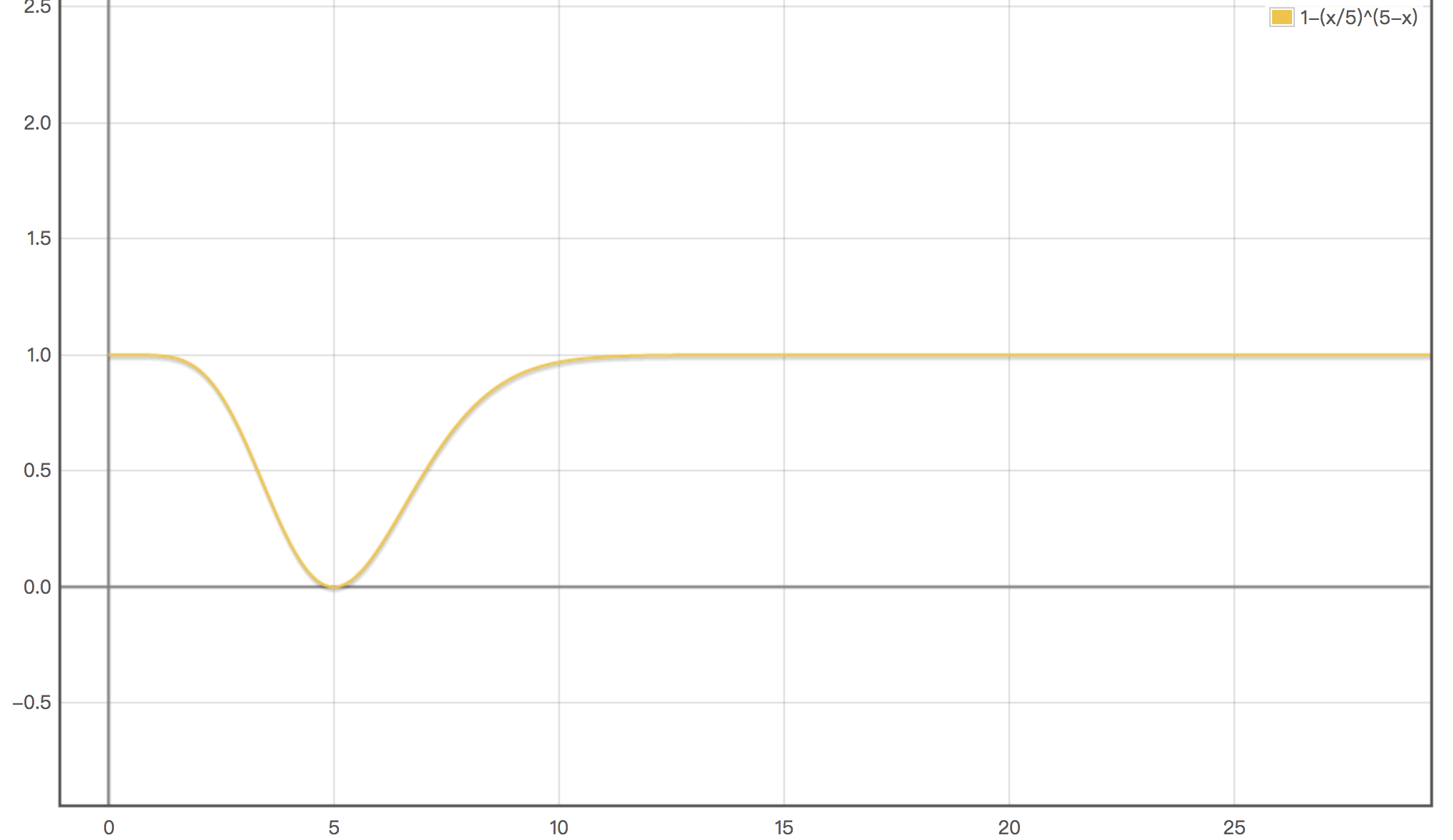### feature maps

pooling完了后，接的是tanh。本文里把经过了卷积、pooling和tanh的结果叫1阶feature map。定义第$$i$$阶feature map为$$F^i$$。对于中间的某一层，里面其实有很多个feature map，完全可以并行计算。例如，定义$$F^i_j$$$$i$$阶feature maps里的第$$j$$个feature map，是通过如下方式计算的：将distinct的权重矩阵$$w^i_{j,k}$$和低阶$$i-1$$的每个feature map $$F^{i-1}_k$$的卷积结果加起来：

$F^i_j=\sum ^{m_i}_{k=1}w^i_{j,k}* F^{i-1}_j$

### ccpm小结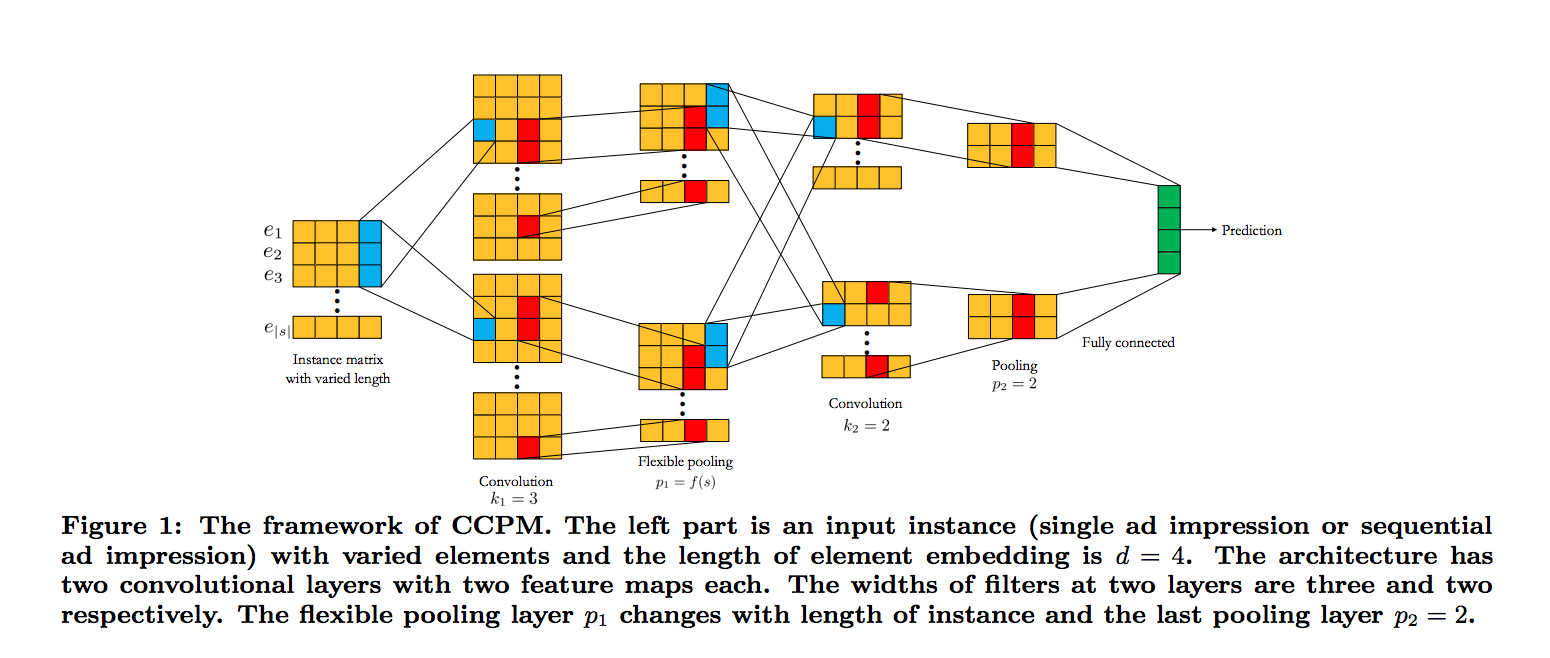## Wide & Deep

Wide & deep learning for recommender systems

LR 对于 DNN 模型的优势是对大规模稀疏特征的容纳能力，包括内存和计算量等方面，工业界都有非常成熟的优化方法； 而 DNN 模型具有自己学习新特征的能力，一定程度上能够提升特征使用的效率， 这使得 DNN 模型在同样规模特征的情况下，更有可能达到更好的学习效果。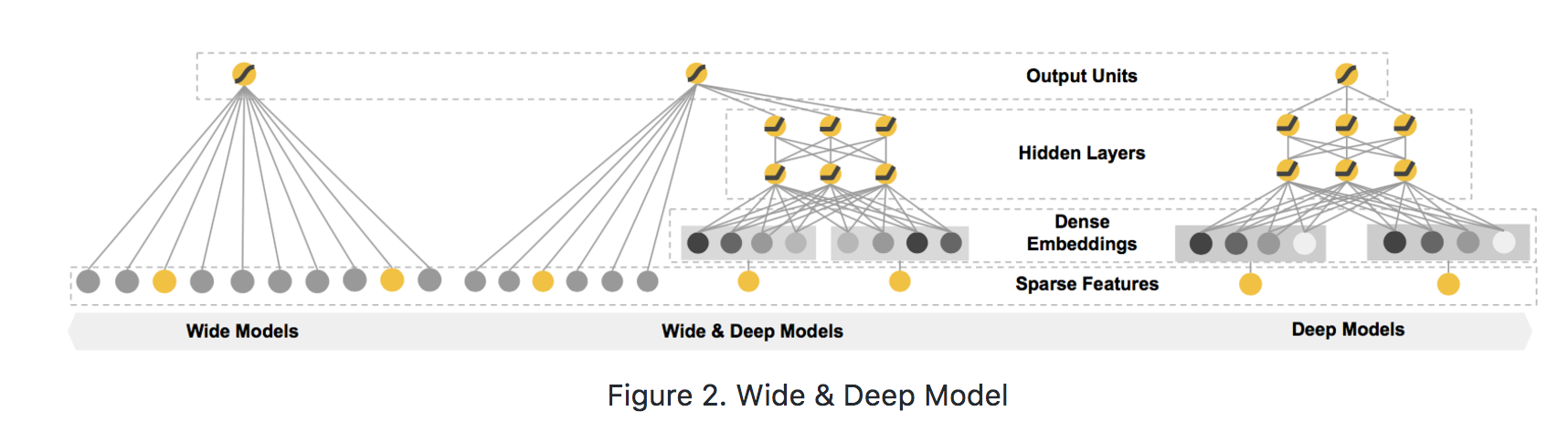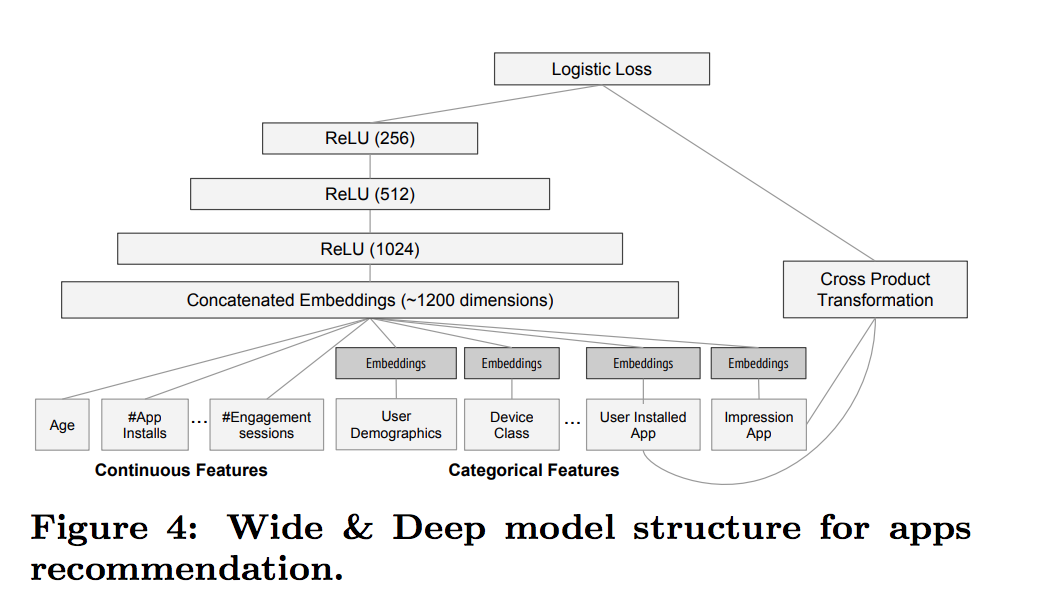• wide侧：用户安装的app和浏览过的app的cross-product
• deep侧：每个categorical产出一个32维的emb，然后concate到一起，再和连续值特征concate到一起得到一个约1200维的vec，再接3层fc和relu(1024->512->256)
• wide和deep的输出加起来（如果是2分类问题，wide和deep的输出就都是一维，如果是n分类问题，那就是n维），加完的结果再丢给sigmoid或者softmax，去和label算交叉熵

tf代码：

def get_model(model_type, model_dir):
print("Model directory = %s" % model_dir)

# 对checkpoint去做设定
runconfig = tf.contrib.learn.RunConfig(
save_checkpoints_secs=None,
save_checkpoints_steps = 100,
)

m = None

# 宽模型
if model_type == 'WIDE':
m = tf.contrib.learn.LinearClassifier(
model_dir=model_dir,
feature_columns=wide_columns)

# 深度模型
if model_type == 'DEEP':
m = tf.contrib.learn.DNNClassifier(
model_dir=model_dir,
feature_columns=deep_columns,
hidden_units=[100, 50, 25])

# 宽度深度模型
if model_type == 'WIDE_AND_DEEP':
m = tf.contrib.learn.DNNLinearCombinedClassifier(
model_dir=model_dir,
linear_feature_columns=wide_columns,
dnn_feature_columns=deep_columns,
dnn_hidden_units=[100, 70, 50, 25],
config=runconfig)

print('estimator built')

return m


https://www.tensorflow.org/api_docs/python/tf/estimator/DNNLinearCombinedClassifier

linear_optimizer='Ftrl',


https://stackoverflow.com/questions/34945554/how-to-set-layer-wise-learning-rate-in-tensorflow

## DeepFM

DeepFM: A Factorization-Machine based Neural Network for CTR Prediction

DeepFM和之前模型相比优势在于两点:

• 相对于Wide&Deep不再需要手工构建wide部分
• 另一个相对于FNN，FNN把FM的隐向量参数直接作为网络参数学习。而DeepFM将embedding层结果输入给FM和MLP，两者输出叠加，达到捕捉了低阶和高阶特征交叉的目的。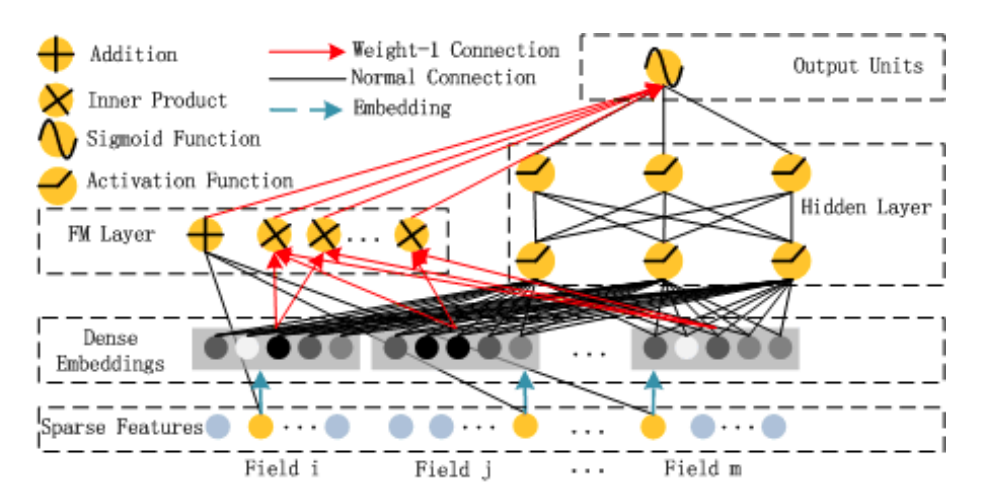## Deep & Cross

DCN的特点如下：

• Deep部分就是普通的MLP网络，主要是全连接。
• 与DeepFM类似，DCN是由embedding+MLP部分与cross部分进行联合训练的。Cross部分是对FM部分的推广。
• 可以证明，cross网络是FM的过程在高阶特征组合推广
• 只有两层，且第一层最后一层权重参数相等时的Cross网络与简化版FM等价
• 简化版的FM指的是，将拼接好的稠密向量作为输入向量，且不做领域方面的区分（但产生这些稠密向量的过程是考虑领域信息的，相对全特征维度的全连接层减少了大量参数，可以视作稀疏链接思想的体现）。而且之后进行embedding权重矩阵W只有一列——是退化成列向量的情形
• 与MLP网络相比，Cross部分在增加高阶特征组合的同时减少了参数的个数，并省去了非线性激活函数。

## xDeepFM

xDeepFM: Combining Explicit and Implicit Feature Interactions for Recommender Systems

• 重要的特征都是与应用场景息息相关的，针对每一种应用场景，工程师们都需要首先花费大量时间和精力深入了解数据的规律之后才能设计、提取出高效的高阶交叉特征，因此人力成本高昂
• 原始数据中往往包含大量稀疏的特征，例如用户和物品的ID，交叉特征的维度空间是原始特征维度的乘积，因此很容易带来维度灾难的问题
• 人工提取的交叉特征无法泛化到未曾在训练样本中出现过的模式中。

### 背景

FM将每个feature映射成一个D维的隐向量$$v_i=[v_{i1},v_{i2},...,v_{iD}]$$，然后将pairwise的特征交叉建模为隐向量的内积$$f^{(2)}(i,j)=\left \langle v_i,v_j \right \rangle x_i x_j$$，本文中将隐向量$$v_i$$的一个元素（例如$$v_{i1}$$）称作『bit』。。传统FM能扩展到任意高阶的特征交互，但同时对有用和无用的组合都建模了。而这些无用的组合可能会引入噪音并降低精度。

FNN（ Factorisation-machine supported Neural Network）在使用DNN之前，为field embedding使用pre-trained的FM。

PNN为embedding layer和DNN layer引入了product layer，从而不需要对pretrained FM的依赖。

FNN和PNN的缺点是，他们更多地关注高阶特征组合，而忽视了低阶的特征组合。

wide&deep和deepFM使用混合架构，在网络中有shallow part和deep part，保证memorization和generalization，从而克服了这个问题。

### 预备知识

#### embedding

$e=[e_1,e_2,...,e_m]$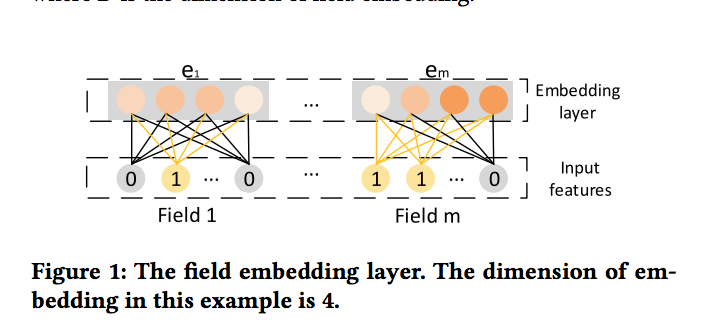#### 隐式的高阶特征交互

FNN，Deep crossing，wide&deep的deep部分都是直接用DNN来隐式地进行高阶特征交互。这里的交互是bit-wise的，也就是说，即使是同一个field里的feature，也会互相影响

• PNN将product layer的输出直接做为DNN的输入
• DeepFM将FM layer直接连到了output unit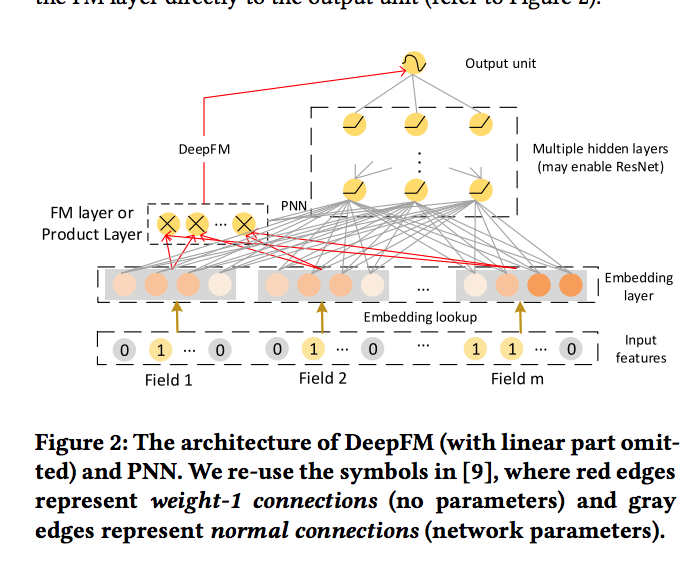#### 显式的高阶特征交互

cross network使用如下公式进行高阶特征组合：

$x_k=x_0x^T_{k-1}w_k+b_k+x_{k-1}$

k=1时，

\begin{align*} x_1 &= x_0(x_0)^Tw_1+x_0 \\ &=x_0(x_0^Tw_1+1) \\ &=\alpha ^1x_0 \end{align*}

scalar$$\alpha ^1$$$$x_0$$的一个线性变换($$x_0$$是dx1，所以$$x_0^T$$是1xd，而$$w_1$$是dx1，所以$$x_0^Tw_1$$是一个标量。。然后假设k=i成立，即$$x_i=\alpha ^i x_0$$。对于k=i+1，

\begin{align*} x_{i+1} &= x_0(x_i)^Tw_{i+1}+x_i \\ &=x_0((\alpha ^ix_0)^Tw_1)+ \alpha ^ix_0 \\ &=\alpha ^{i+1}x_0 \end{align*}

• 每一层变成了$$x_0$$与一个标量相乘
• 高阶的特征交互变成了bit-wise，而不是FM所期待的vector-wise### CIN

$X^k_{h,*}=\sum ^{H_{k-1}}_{i=1}\sum ^{m}_{j=1}W^{k,h}_{ij}(X^{k-1}_{i,*}\circ X^{0}_{j,*})$

• 根据前一层隐层的状态$$X^k$$和原特征矩阵$$X^0$$，计算出一个中间结果$$Z^{k+1}$$，它是一个三维的张量。注意图中的$$\bigotimes$$是outer product，其实就是矩阵乘法咯，也就是一个mx1和一个nx1的向量的外积是一个mxn的矩阵：

$u\bigotimes v=uv^T=\begin{bmatrix} u_1\\ u_2\\ u_3\\ u_4 \end{bmatrix}\begin{bmatrix} v_1 & v_2 & v_3 \end{bmatrix}=\begin{bmatrix} u_1v_1 & u_1v_2& u_1v_3 \\ u_2v_1 & u_2v_2 & u_2v_3\\ u_3v_1 & u_3v_2 & u_3v_3\\ u_4v_1 & u_4v_2 & u_4v_3 \end{bmatrix}$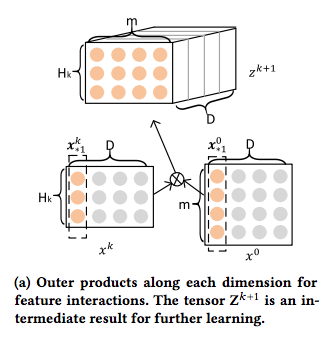• 接下来，如下图所示：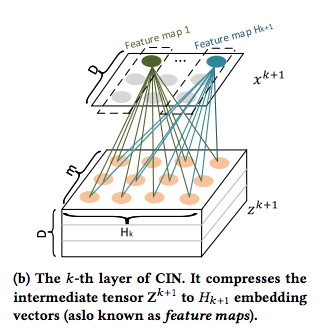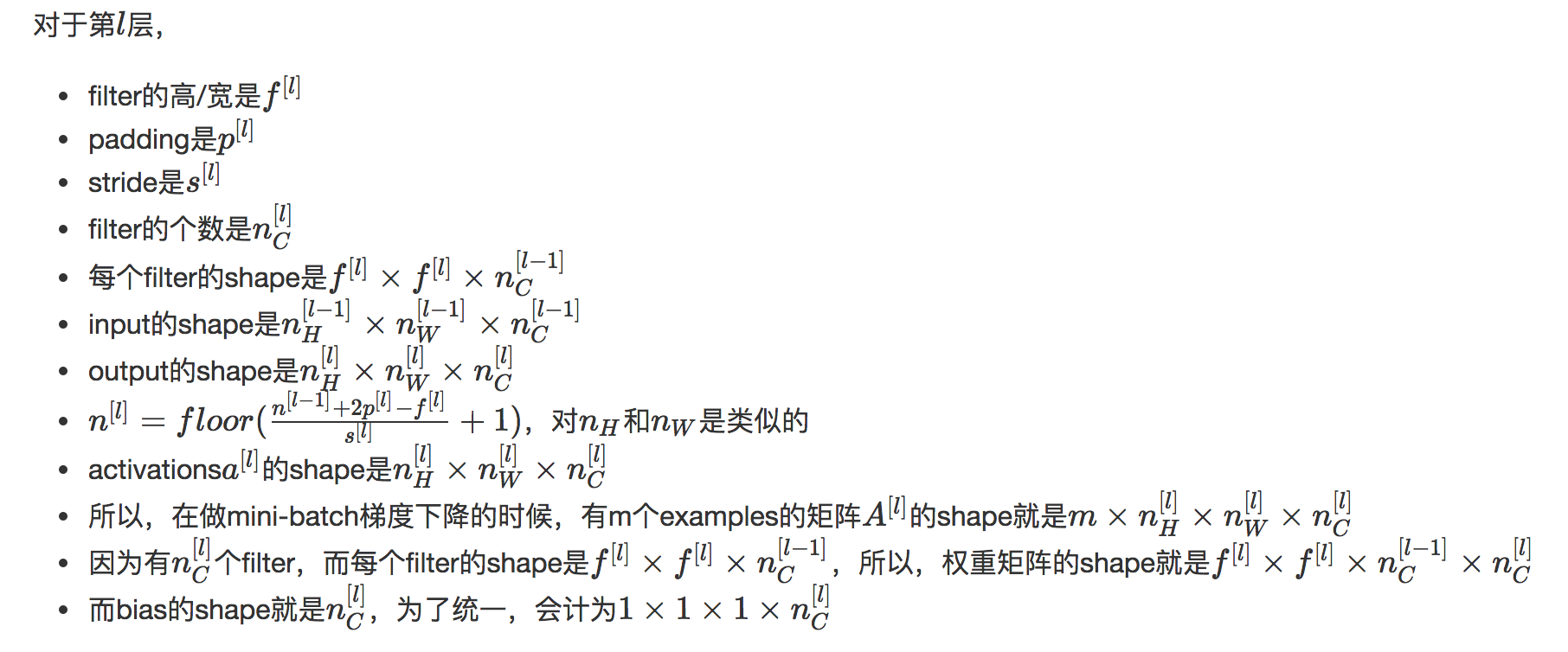split_tensor = tf.split(hidden_nn_layers[-1], hparams.dim * , 2)
dot_result_m = tf.matmul(split_tensor0, split_tensor, transpose_b=True)
dot_result_o = tf.reshape(dot_result_m, shape=[hparams.dim, -1, field_nums*field_nums[-1]])
dot_result = tf.transpose(dot_result_o, perm=[1, 0, 2])

filters = tf.get_variable(name="f_"+str(idx),
shape=[1, field_nums[-1]*field_nums, layer_size],
dtype=tf.float32)
# dot_result = tf.transpose(dot_result, perm=[0, 2, 1])
curr_out = tf.nn.conv1d(dot_result, filters=filters, stride=1, padding='VALID')


CIN的总体框架如下图：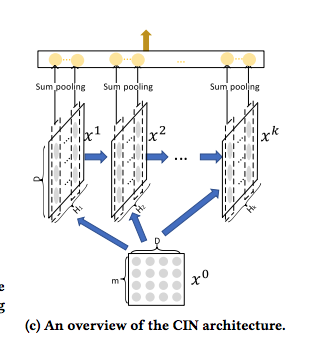CIN的结构与RNN是很类似的，即每一层的状态是由前一层隐层的值与一个额外的输入数据计算所得。不同的是，

• CIN中不同层的参数是不一样的，而在RNN中是相同的；
• RNN中每次额外的输入数据是不一样的，而CIN中额外的输入数据是固定的，始终是$$X^0$$

### xDeepFM

CIN+DNN+linear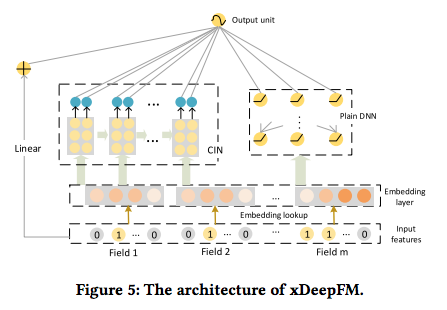## DIN

Deep Interest Network for Click-Through Rate Prediction

• 用户的每个领域的历史特征权重则由该历史特征及其对应备选广告特征通过一个子网络得到。即用户历史浏览的商户特征当前浏览商户特征对应，历史浏览的商品特征当前浏览商品特征对应。
• 权重子网络主要包括特征之间的元素级别的乘法、加法和全连接等操作。
• AFM也引入了注意力机制。但是AFM是将注意力机制与FM同领域特征求和之后进行结合，DIN直接是将注意力机制与同领域特征求和之前进行结合。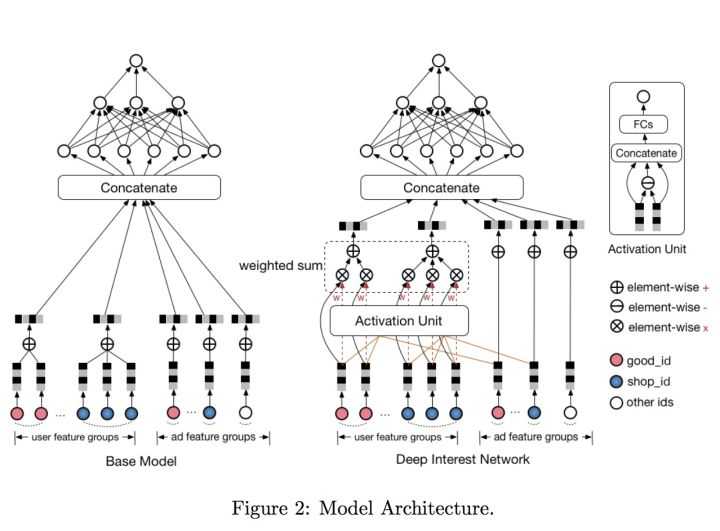## DIEN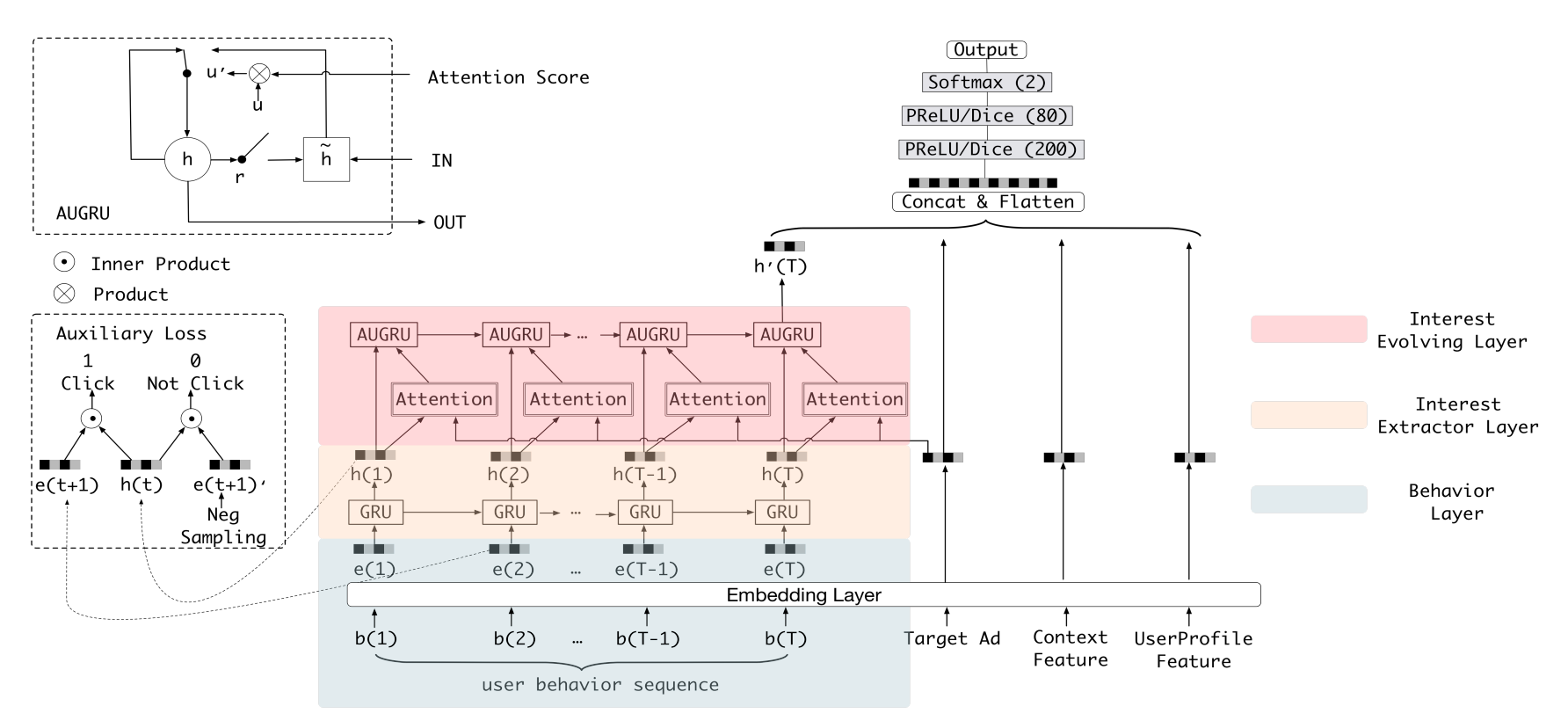### 兴趣提取

• 一方面需要和下一个时间步输入用户点击历史里点击样本$$e(t+1)$$去算一个点击的loss
• 另一方面，需要和下一个时间步输入负采样样本$$e'(t+1)$$去算另一个不点击的loss

### 兴趣演化

$\begin{matrix} r_t=\sigma(W^ri_t+U^rh_{t-1}+b^r) \\ u_t=\sigma(W^ui_t+U^uh_{t-1}+b^u) \\ u'_t=u_t*a_t \\ \hat{h_t}=tanh(W^hi_t+r_t\circ U^hh_{t-1}+b^h)\\ h_t=(1-u'_t)\circ h_{t-1}+u'_t\circ \hat{h_t} \end{matrix}$

$\\ z_t=\sigma(W_zx_t+U_zh_{t-1}+b_z) \\ r_t=\sigma(W_rx_t+U_rh_{t-1}+b_r) \\ h_t=z_t \circ h_{t-1}+(1-z_t) \circ tanh(W_hx_t+ U_h(r_t \circ h_{t-1}) + b_h)$

$$a_t$$是ad和当前时间点兴趣(由兴趣提取层提取得到)的相关度权重

$a_i=\frac{exp(h_iWe_{ad})}{\sum^T_{i=0}exp(h_jWe_{ad})}$

https://github.com/alibaba/x-deeplearning/blob/master/xdl-algorithm-solution/DIEN/script/utils.py#L139

## ESMM

Entire Space Multi-Task Model: An Effective Approach for Estimating Post-Click Conversion Rate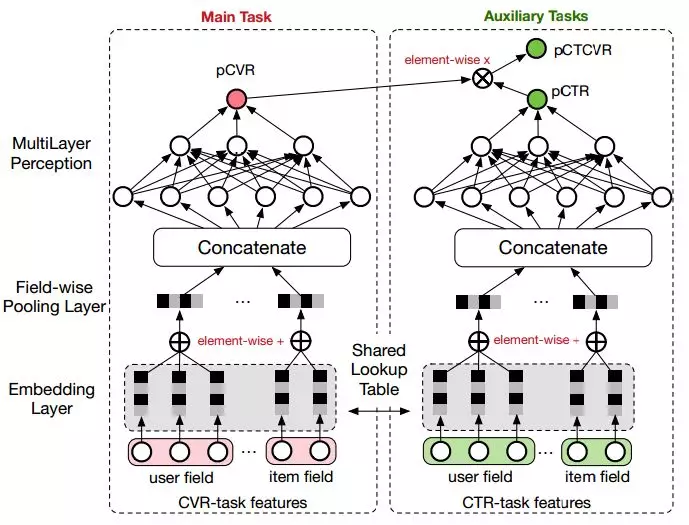$L(\theta_{cvr},\theta_{ctr})=\sum ^N_{i=1}l(y_i,f(x_i;\theta_{ctr}))+\sum ^N_{i=1}l(y_i\&z_i,f(x_i;\theta_{ctr})\times f(x_i;\theta_{cvr}))$

ESMM模型是在整个样本空间建模，而不像传统CVR预估模型那样只在点击样本空间建模。

## TDM

Learning Tree-based Deep Model for Recommender Systems

https://github.com/alibaba/x-deeplearning/wiki/%E6%B7%B1%E5%BA%A6%E6%A0%91%E5%8C%B9%E9%85%8D%E6%A8%A1%E5%9E%8B(TDM)

CIKM 2019 EComm AI：超大规模推荐之用户兴趣高效检索 赛题解读及阿里深度树匹配技术实践

## DeepGBM

DeepGBM 主要包含两个子模块——面向类别特征的 CatNN 和面向数值特征的 GBDT2NN。CatNN 主要继承了 NN 中对类别特征友好的 Deep 模块和 FM 模块；GBDT2NN 是文章最主要的贡献之一，其基于 GBDT 进行知识蒸馏构造了一个 NN 模块，可以有效地处理类别特征并可以在线更新。综上，DeepGBM 既支持含有类别特征和数值特征的表格型数据输入，还能利用实时产生的数据进行学习和更新。

## 各种比赛

2019腾讯广告大赛：

https://zhuanlan.zhihu.com/p/73062485

Key-Value Memory Networks for Directly Reading Documents

comment here..